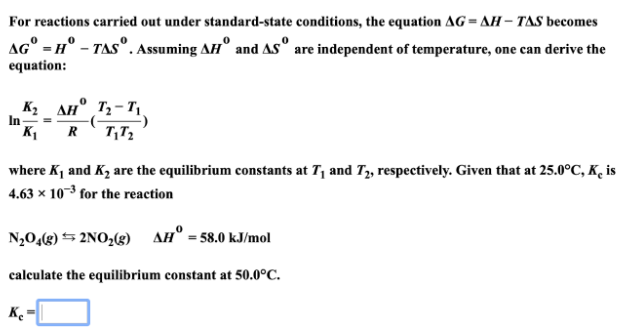# For reactions carried out under standard-state conditions, the equation ΔG = ΔH - TΔS becomes ΔG° = H° - TΔS°. Assuming ΔH° and ΔS° are independent of temperature, one can derive the equation: where K1 and K2 are equilibrium constants at T1 and T2, respectively. Given that at 25.0°C, Kc is 4.63 x 10^-3 for the reaction N2O(g) ⇋ 2NO2(g) ΔH° = 58.0 kJ/mol calculate the equilibrium constant of 50.0°C.